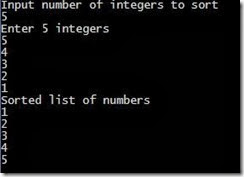## Coldfusion Java JQuery

An online resource for latest web technologies like Coldfusion, JRun, Pro*C, JQuery, HTML5, PHP, W3C, Java, J2EE, C, C++, ORACLE, PL/SQL, MySql, Ajax, Coldbox, Fusebox, UNIX, JavaScript, NodeJS and much more...

## Wednesday, February 26, 2014

### Bubble sort in java

The logic is to swap the 2 items in the list during iterations. At the end of first iteration the list will have the largest no. at the end.

n-1 iterations for n items. Time complexity is O(n2)

`import java.util.Scanner; class BubbleSort {  public static void main(String []args) {    int n, c, d, swap;    Scanner in = new Scanner(System.in);     System.out.println("Input number of integers to sort");    n = in.nextInt();     int array[] = new int[n];     System.out.println("Enter " + n + " integers");     for (c = 0; c < n; c++)       array[c] = in.nextInt();     for (c = 0; c < ( n - 1 ); c++) {      for (d = 0; d < n - c - 1; d++) {        if (array[d] > array[d+1]) /* For descending order use < */        {          swap       = array[d];          array[d]   = array[d+1];          array[d+1] = swap;        }      }    }     System.out.println("Sorted list of numbers");     for (c = 0; c < n; c++)       System.out.println(array[c]);  }}`

Output will be like: